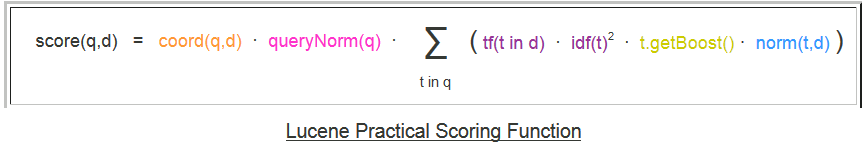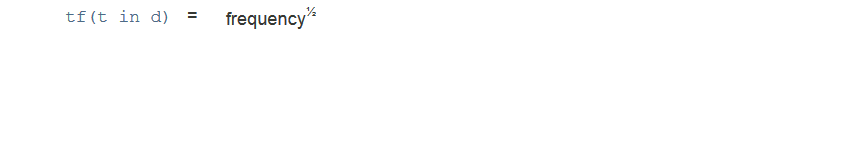# Understanding Solr Explain

When we search documents on solr, the documents in the result are in descending order of their scores. If we want to understand how the score of document in the result is calculated then, in the solr query add &debugQuery=true. Solr explain part, will be appended in the solr result after adding &debugQuery=true in the solr query.

For example:

select?q=summary:”Apache solr”&fl=id,summary,score &debugQuery=true

When I executed above query on solr, I got result as shown below:

`<result name="response" numFound="2" start="0" maxScore="0.2972674">    <doc>        <str name="id">978-0641723445</str>        <str name="summary">Solr in Action is a comprehensive guide to implementing scalable search using Apache Solr. </str>        <float name="score">0.2972674</float>    </doc>    <doc>        <str name="id">978-1423103349</str>        <str name="summary">Apache Solr 4 Cookbook is written in a helpful, practical style with numerous hands-on recipes to help you master Apache Solr to get more precise search results and analysis, higher performance, and reliability. This book is for developers who wish to learn how to master Apache Solr 4. This book will specifically appeal to developers who wish to quickly get to grips with the changes and new features of Apache Solr 4. This book is also handy as a practical guide to solving common problems and issues when using Apache Solr.</str>        <float name="score">0.24926631</float>    </doc></result>`

The above query returned 2 documents which are ranked in descending order of their score :

First document with id=978-0641723445 and score=0.25373363

Second document with id=978-1423103349 and score=0.17625791

The document (id=978-0641723445) has “Apache Solr” phrase 1 times in summary field where as document (id=978-1423103349) has “Apache Solr” phrase 5 times in summary field, but still the document (id=978-1423103349) score is less than document (id=978-0641723445).

We will try to understand reason for this.

The calculated score not only depends upon how many times search query string is present in searched field, but it also depends upon other factors as explained in Lucene’s TFIDFSimilarity.The score of the document is calculated based on above formula.

Not lets looks at the Explain part present in the solr result:

` <lst name="explain">    <str name="978-0641723445">0.2972674 = (MATCH) weight(summary:"apache solr" in 0) [DefaultSimilarity], result of:  0.2972674 = score(doc=0,freq=1.0 = phraseFreq=1.0), product of:    0.99999994 = queryWeight, product of:      1.1890697 = idf(), sum of:        0.5945349 = idf(docFreq=2, maxDocs=2)        0.5945349 = idf(docFreq=2, maxDocs=2)      0.8409935 = queryNorm    0.29726744 = fieldWeight in 0, product of:      1.0 = tf(freq=1.0), with freq of:        1.0 = phraseFreq=1.0      1.1890697 = idf(), sum of:        0.5945349 = idf(docFreq=2, maxDocs=2)        0.5945349 = idf(docFreq=2, maxDocs=2)      0.25 = fieldNorm(doc=0)</str>    <str name="978-1423103349">0.24926631 = (MATCH) weight(summary:"apache solr" in 0) [DefaultSimilarity], result of:  0.24926631 = score(doc=0,freq=5.0 = phraseFreq=5.0), product of:    0.99999994 = queryWeight, product of:      1.1890697 = idf(), sum of:        0.5945349 = idf(docFreq=2, maxDocs=2)        0.5945349 = idf(docFreq=2, maxDocs=2)      0.8409935 = queryNorm    0.24926633 = fieldWeight in 0, product of:      2.236068 = tf(freq=5.0), with freq of:        5.0 = phraseFreq=5.0      1.1890697 = idf(), sum of:        0.5945349 = idf(docFreq=2, maxDocs=2)        0.5945349 = idf(docFreq=2, maxDocs=2)      0.09375 = fieldNorm(doc=0)</str>  </lst> `

Lets try to understand, how the score is calculated.

idf(t):

I have indexed only 2 documents and terms in phrase “Apache solr” are present in summary field of both document

So for term “Apache” docFreq=2, maxDocs=2

Similarly for term “solr” docFreq=2, maxDocs=2

So based on the formula as given below,For term “Apache” idf=0.5945349

For term “solr” idf=0.5945349

Thus the idf calculated is same for both documents.

tf(t in d):

Now “Apache solr” phrase is present 1 times in document (978-0641723445) and 5 times in document (978-1423103349)

So based on the formula as given below.For document (978-0641723445) : tf(phraseFreq==1.0) = 1

For document (978-1423103349) : tf(phraseFreq=5.0) = 2.236068

queryNorm:

As all terms in query are present in both documents,calculated queryNorm is same for both document (0.8409935 = queryNorm)

fieldNorm :

One of the factor for calculating fieldNorm is the length of text in the searched field. Shorter the field text length (Number of terms in field) higher is the score.

Since text length of summary field in document (978-0641723445) is less than text length of summary field in document (978-1423103349)

For document (978-0641723445) fieldNorm=0.25

And for document (978-1423103349) fieldNorm=0.09375

Thus due to calculated fieldNorm the final score of document (978-0641723445) is higher than document (978-1423103349).